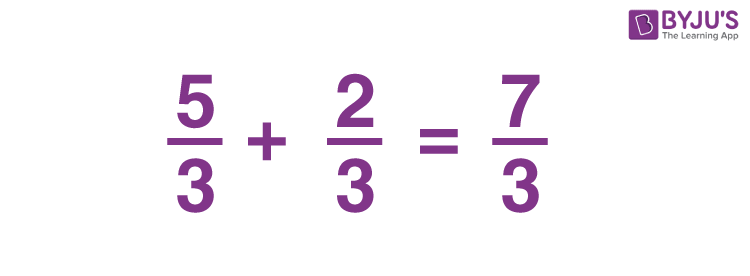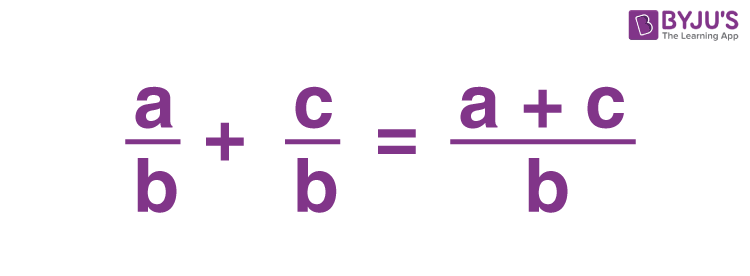The addition of fractions teaches us to add two or more fractions with the same or different denominators. The addition of fraction depends on two major conditions:

• Same denominators
• Different denominatorsWhile adding fractions, if denominators are the same (such fractions are said to be like fractions), then they can be added directly. But if the denominators are different, (such fractions are called unlike fractions) then we need to make the denominators same and then add the fractions. Learn like or unlike fractions, here.

## Addition of Fractions with Same Denominators

If denominators of two or more fractions are same, then we can directly add the numerators, keeping the denominator common.

• Add the numerators together, keeping the denominator common
• Write the simplified fractionFor example: Add the fractions: 5/6 and 7/6.

Since the denominators are same, therefore we can add the numerators directly.

(5/6) + (7/6) = (5 + 7)/6 = 12/6

Simplify the fraction

12/6 = 2

Hence, the sum of ⅚ and 7/6 is 2.

## Adding fractions with Different Denominators

When two or more fractions with different denominators are added together, then we cannot the numerators directly.

• Check the denominators of the fractions.
• Make the denominators of the fractions same, by finding the LCM of denominators and rationalising them
• Add the numerators of the fractions, keeping the denominator common
• Simplify the fraction to get final sum

For example: Add 3/12 + 5/2

Solution: Both the fractions 3/12 and 5/2 have different denominators.

We can write 3/12 = ¼, in a simplified fraction.

Now, ¼ and 5/2 are two fractions.

LCM of 2 and 4 = 4

Multiply 5/2 by 2/2.

5/2 x 2/2 = 10/4

¼ + 10/4 = (1+10)/4 = 11/4

Hence, the sum of 3/12 and 5/2 is 11/4.

## Adding fractions with whole numbers

Add the fraction and a whole number with three simple steps:

• Write the given whole number in the form of a fraction (for example, 3/1)
• Make the denominators same and add the fractions
• Simplify the fraction

For example: Add 7/2 + 4

Here, 7/2 is a fraction and 4 is a whole number.

We can write 4 as 4/1.

Now making the denominators same, we get;

7/2 and 4/1 x (2/2) = 8/2

7/2 + 8/2 = 15/2

Hence, the sum of 7/2 and 4 is 15/2.

## Adding Fractions with Co-prime Denominators

Co-prime denominators: The denominators which do not have common factors, other than 1.

Let us learn how to add fractions with co-prime denominators with the help of the following steps:

• Check the denominators whether they are co-prime
• Multiply the first fraction (numerator and denominator) with the denominator of the other fraction and the second fraction (numerator and denominator) with the denominator of the first fraction.
• Add the resulting fractions and simplify

For example, the addition of fractions 9/7 and 3/4 can be done as follows.

The denominators 7 and 4 are coprime since they have only one highest common factor 1.

So, (9/7) + (3/4) = [(9 × 4) + (3 × 7)]/ (7 × 4)

= (36 + 21)/28

= 57/28

A mixed fraction is a combination of a whole number and a fraction. To add two mixed fractions, we need to convert them first into improper fractions and then add them together.

• Convert the given mixed fraction into improper fractions
• Check if denominators are the same or different
• If different denominators, then rationalise them
• Add the fractions and simplify

Let’s understand how to add mixed fractions with an example:

Example: Add : 3 ⅓  + 1 ¾

Solution:

Step1: Convert the given mixed fractions to improper fractions.

3 ⅓  = 10/3

1 ¾ = 7/4

Step 2: Make the denominators same by taking the LCM and multiplying the suitables fractions for both.

LCM of 3 and 4 is 12.

So, 10/3 = (10/3) × (4/4) = 40/12

7/4 = (7/4) × (3/3) = 21/12

Step 3: Take the denominator as common and add numerators.  Then, write the final answer.

(40/12) + (21/12) = (40 + 21)/12 = 61/12

Therefore, 3 ⅓  + 1 ¾ = 61/12 = 5 1/12

## Subtraction of Fractions

As we know, addition and subtraction are similar operations in Maths. In addition, we add two or more numbers, whereas, in subtraction, we subtract a number from another. Therefore, subtraction of fractions also follows the same rule as addition of fractions.

If the denominators are the same for given fractions, then we can directly subtract the numerator, keeping the denominator same.

If the denominators of fractions are different, we need to rationalise them first and then perform subtraction.

Some examples are:

Example 1: Subtract ⅓ from 8/3.

Solution: We need to find,

8/3 – ⅓ = ?

Since the denominator of two fractions ⅓ and 8/3 is common, therefore, we can directly subtract them:

8/3 – ⅓ = (8-1)/3 = 7/3

Example 2: Subtract ½ from ¾.

Solution: We need to subtract ½ from ¾, i.e.,

¾ – ½ = ?

Since the denominators of two fractions are different, therefore, we need to rationalise them by taking the LCM.

LCM (4,2) = 4

Now multiply the ½ by 2/2, to get 2/4

Therefore,

¾ – 2/4 = (3-2)/4 = ¼

Hence, ¾ – ½ = ¼

## Video Lesson on Fractions## Solved Examples

Let us solve some problems based on adding fractions.

Q. 1: Add 1/2 and 7/2.

Solution: Given fractions: 1/2 and 7/2
Since the denominators are the same, hence we can just add the numerators here, keeping the denominator as it is.

Therefore,

1/2 + 7/2

= (1+7)/2

= 8/2

=4

Q. 2: Add 3/5 and 4.

Solution: We can write 4 as 4/1

Now, 3/5 and 4/1 are the two fractions to be added.

Since the denominators here are different, thus we need to simplify the denominators first, before adding the fractions.

Hence,

3/5 + 4/1

Taking LCM of 5 and 1, we get;

LCM(5,1) = 5

Therefore, multiplying the second fraction, 4/1 by 5 both in numerator and denominator, we get;

(4×5)/((1×5) = 20/5

Now 3/5 and 20/5 have a common denominator, i.e. 5, therefore, adding the fractions now;

3/5 + 20/5

= 23/5

Fraction addition is one of the important topics in classes 6, 7 and 8. We have provided a worksheet for the addition of fractions here. After practising the questions given in this worksheet, you’ll be able to solve ant fraction addition sums easily. Practice from the given addition of fraction worksheet link here and score well in exams.

## Practice Questions

1. ⅜ + ⅝ =
2. 1(⅓) + 3(5/2) =
3. 2(¾) + ___ = 7
4. ⅖ + ⅔ =
5. 3/7 + 2 + 4/3 = ?

## Related Articles

Visit BYJU’S to learn other mathematical topics in an interesting way.

## Frequently Asked Questions – FAQs

Q1

### How to add two fractions with different denominators?

To add two fractions, with different denominators, we need to rationalise the denominators by taking out the LCM and make the denominator same. Then add the numerators of the fractions, keeping the denominator common. For example, ½ + ⅗
LCM of 2 and 5 = 10
= (5/5) x (½) + (⅗) x (2/2)
= (5+6)/10
= 11/10
Q2

### What are the rules to add and subtract fractions?

There are two simple rules to add and subtract fractions. If the denominators are the same, then we can directly add and subtract fractions. But if denominators are different then we need to rationalise the denominators by finding LCM of the two and then add the fractions.
Q3

### How to add whole numbers and fractions?

If we add a whole number and a fraction, then we need to first write the whole number in the form of fraction. For example, adding 3 and ½ we get,
3+½ = 3x(2/2) + ½ = 6/2 + ½ = 7/2
Q4

### How to add large fractions?

Let us take two fractions: 11/24 and 9/60
LCM of 24 and 60 = 120
Therefore,
= (11/24)x(5/5) + (9/60)x(2/2)
= (55+18)/120
= 73/120
Q5

### How to add fractions with like denominators?

Let us say, ⅗ and 7/5 are two fractions. Since ⅗ and 7/5 are two like fractions, having same denominator, we can add the numerators directly.
Therefore,
⅗ + 7/5 = (3+7)/5 = 10/5 = 2